Math 421 diary, fall 2005 Fourier series

 Confession

Thursday, November 10

 Fourier series formulas
Textbook problem presentations
Many people presented problems from sections 12.1 and 12.2, and I hope to get the writeups and scan them and post them on the web. As I mentioned, I hope that most of the time you will be able to use a calculator or computer to get the Fourier coefficients of a function. What can't yet be obtained from a computer is the behavior of the whole series and "high" partial sums of the series compared to the original function.

We had more fun with Fourier series. I reviewed the formulas for Fourier coefficients. I wrote also how these were used to assemble the Fourier series for a function. I noted that if a function f were periodic with period 2Pi, then any interval of length 2Pi will be good for computing the Fourier coefficients. So, for example, if I wanted a14, what I wrote last time is (1/Pi)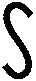02Pif(x)cos(14x) dx and the textbook has (1/Pi)-PiPif(x)cos(14x) dx. But if for some peculiar reason you wanted to use (1/Pi)668668+2Pif(x)cos(14x) dx you would get the same answer. Of course, for this, you should realize that the function f(x) must be periodic with period equal to 2Pi.

Now what should we expect about the Fourier series of f(x)? I really tried to think about the levels of information engineering students should know about Fourier series.

Primary level
(What you really need to know)
On average, if you look at a "high" partial sum of the Fourier series for f, then random samples of the values of this partial sum will be close to the values of f(x). A precise statement is that the mean square error will --> 0 as more terms are taken of the partial sums.

Secondary level
(What you should know for Math 421)
The sum of whole Fourier series for a function, f, will be f(x) if f is continuous at x. If f has a jump discontinuity at x, then the sum of the whole Fourier series will be (f(x-)+f(x+))/2, the average of the left and right hand limits of f. Notice, though, that from the point of view of Fourier series, 0 and 2Pi are the same, so the left side of 0 is the left side of 2Pi, and the right side of 0 is the right side of 2Pi. Or, if you are considering the interval [-Pi,Pi]. the whole Fourier series and the partial sums think that -Pi and Pi are the same.
Comment This property really isn't just for 421, but may also be useful in applications: I may be exaggerating about my classifications!

Tertiary level
(What Fourier series enthusiasts might know)
The Gibbs phenomenon: if f has a jump discontinuity at x, then the partial sums exhibit over and undershoots very near the jump (about 9% of the jump in directions opposite the jump), and the bumps are opposite direction of the jump.
Notice, please that the sum of the whole Fourier series does not does not have this behavior. Its behavior was described above. I remarked that I did know of some real-world applications where this Gibbs phenomenon was important, but I didn't know very many.

I went through the graphical behavior implicit in some of the homework problems. The alert student should be able to see the phenomena described above in these pictures. Actually computing the Fourier series in each case because (if this is being done "by hand") in each case the Fourier coefficients need two integrations by parts.

F(x)=ex on [-Pi,Pi]
The function itself The partial sum, up to n=10,
of the Fourier series
The sum of the whole Fourier series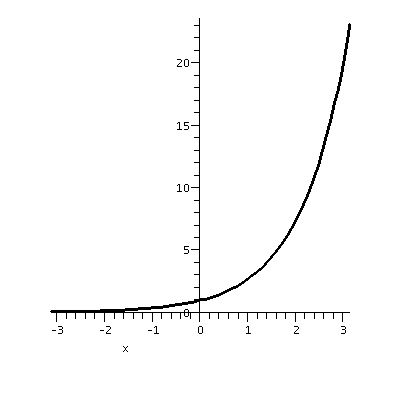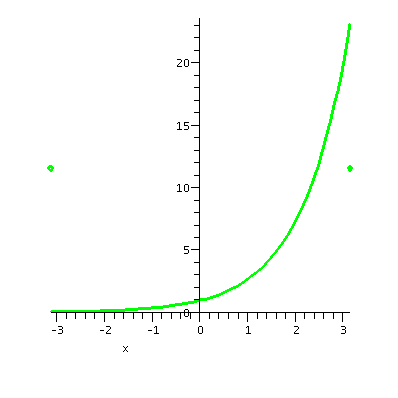F(x)=x2 on [0,Pi] and 0 on [-Pi,0]
The function itself The partial sum, up to n=10,
of the Fourier series
The sum of the whole Fourier series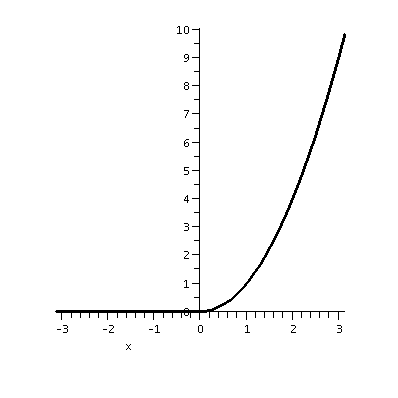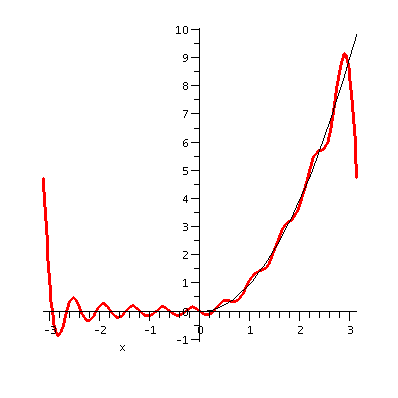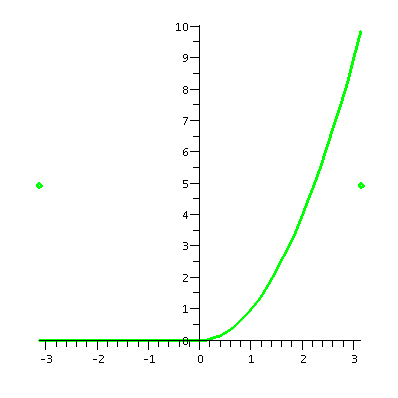Example 1
Suppose f(x)=5sin(x)-2cos(3x)+8cos(17x). What is the Fourier series of f(x)? This is a very cute problem. The Fourier series of f(x) is ...5sin(x)-2cos(3x)+8cos(17x). It is its own Fourier series. Why is that? Any other sine/cosine coefficient would be gotten by integrating (the an or bn formulas). But the other sine/cosine functions are all orthogonal to these. So, for example, a17 is gotten by multiplying f(x) by cos(17x) and integrating from -Pi to Pi. Hey: by orthogonality this is 0. What about a17? Well, by orthogonality you only need to "worry" about (1/Pi)-PiPi8(cos(17x))2dx, and (we discussed this at great length!) this is just 8. The darn 1/Pi in the original formula is included (orthonormalization!) to make the coefficient come out correctly.

Example 2
This example is more computationally intricate, especially when done "by hand". F(x) is defined initially on the interval [0,Pi]. It is piecewise linear, and is the sort of function we encountered in our study of Laplace transform methods. The points (0,0) and (Pi/2,1) and (Pi,1) are on the graph, which suggests that F(x) be (2/Pi)x in the interval 0<x<Pi/2 and F(x)=1 for Pi/2<x<Pi. I "extended" F(x) to be 0 in [-Pi,0]. A Maple expression defining such an F(x) is:
F:=x->piecewise(x>Pi/2,1,x>0,(2/Pi)*x,x>0,0)
I actually computed, by hand, some of the Fourier coefficients. This involved integrating by parts. I hope that students can integrate by parts.

I just asked my friend (?) Maple to do the same computation. The results were:

```The cosine coefficients, a(n):

Pi n
sin(Pi n) Pi n + 2 cos(----) - 2
2
--------------------------------
2
Pi n
The sine coefficients, b(n):

Pi n
-cos(Pi n) Pi n + 2 sin(----)
2
-----------------------------
2
Pi n

a(0);

3
---
8```
The n2's occur because of the integration by parts. I need to evaluate a(0) separately, since I can't just plug in n=0 in a formula with n in the bottom (yes, I could use L'Hopital's rule, but I could also just evaluate the integral). and just for the fun (?) of it, here is the partial sum up to third order, of the Fourier series of F(x):
```        2 cos(x)   (Pi + 2) sin(x)   cos(2 x)       sin(2 x)
3/8 - -------- + --------------- - -------- - 1/2 --------
2              2             2             Pi
Pi             Pi            Pi

cos(3 x)       (3 Pi - 2) sin(3 x)
- 2/9 -------- + 1/9 -------------------
2                    2
Pi                   Pi```
And here is a picture of F(x), a picture of F(x) together with the 10th partial sum of its Fourier series, and a picture of the sum of the whole Fourier series of F(x). You can see that the Fourier series is trying to get close to F(x). On most of the horizontal line segments and on the tilted line, the partial sum of the Fourier series is wiggling above and below. At the endpoints, though, the partial sum wants to have the same value at -Pi and Pi. So the value the partial sum takes is 1/2, the appropriate average of 0 and 1. Also, the Gibbs phenomenon is again visible, if you care about it.

F(x)=0 on [-Pi,0], (2/Pi)x on [0,Pi/2], and 1 on [Pi/2,Pi]
The function itself The partial sum, up to n=10,
of the Fourier series
The sum of the whole Fourier series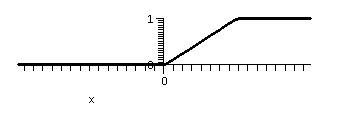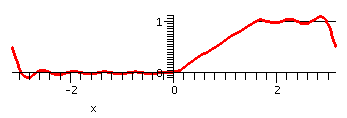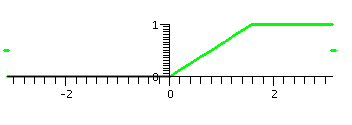The even extension
There are several standard ways of extending a function defined on [0,Pi]. One is the even extension, which asks for a function so that G(-x)=G(x). To get the graph, just flip what you are given across the y-axis. There are some interesting consequences. One is that all of the Fourier sine coefficients are 0. Why? Look at         bn=(1/Pi)-PiPiG(x)sin(nx)dx
When we change x to -x, the integrand, G(x)sin(nx) changes to F(-x)sin(-nx) which is the same as -F(x)sin(nx). Since we're looking at an interval balanced around 0 (from -Pi to Pi) the contribution at x of G(x)sin(nx) is exactly balanced out by -G(x)sin(nx) at -x. So all of the bn's are 0.

I had Maple compute the third partial sum of the Fourier series for the even extension of f. Here it is:

```                    4 cos(x)   2 cos(2 x)       cos(3 x)
3/4 - -------- - ---------- - 4/9 --------
Pi          Pi              Pi
```
You can see why this is called the Fourier cosine series for Fon [0,Pi] although it is really the restriction to [0,Pi] of the Fourier series of the even extension, G, of F.

I also had Maple graph the even extension and some partial sums. The approximation is really good. Here the sum of the whole Fourier series will exactly be equal to the function -- there are no jumps.

F(x)=0 on [-Pi,0], (2/Pi)x on [0,Pi/2], and 1 on [Pi/2,Pi]
The function itself The partial sum, up to n=5,
of the Fourier series
The sum of the whole Fourier series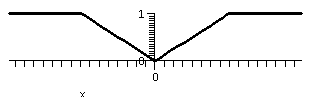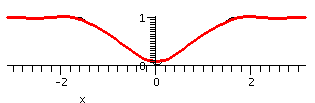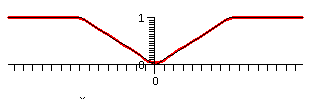The reason I only showed the partial sum up to n=5 above is that the partial sum up to n=10 is amazingly close to the original function. Here it is, to the right. I can't see much of a wiggle, and I can't see much of the original curve at all. It is difficult for me to believe that this is the sum of 10 cosine functions! The sum of the whole Fourier cosine series of F (that is, the Fourier series of the even extension of F) is equal to the original function at all points of [-Pi,Pi].

The odd extension
Now with F defined on [0,Pi] we can extend to a function H(x) defined on [-Pi,Pi] so that H(x)=F(x) on [0,Pi] and H(-x)=-H(x). Flip the graph over the y-axis, and then over the x-axis. Now because         an=(1/Pi)-PiPiH(x)cos(nx)dx
and H(-x)cos(-nx)=-H(x)cos(nx) using the oddness of this extension, we see that all of the an's are 0. Here's the beginning of this Fourier series:

```        2 (Pi + 2) sin(x)   sin(2 x)       (3 Pi - 2) sin(3 x)
----------------- - -------- + 2/9 -------------------
2             Pi                    2
Pi                                  Pi```
Not surprisingly this is called the Fourier sine series for F on [0,Pi] although it is really the restriction to [0,Pi] of the Fourier series of the odd extension, H, of F.

Here are some Maple graphs of the odd extension of together with the sum of the first 10 terms of the Fourier sine series (up to and including the sin(10x) term). The series gets quite close on the tilted line segment, and attempts to be near the two horizontal segments. Of course, there is, in effect, a jump discontinuity at -Pi and Pi. From the Fourier point of view, the odd extension is repeated every 2Pi. So at, for example, x=Pi, the function has a left limit of 1 and a right limit of -1, so the series hops from 1 to -1. To me the Gibbs bumps are showing up.

F(x)=-1 on [-Pi,-Pi/2], (2/Pi)x on [-Pi/2,Pi/2], and 1 on [Pi/2,Pi]
The function itself The partial sum, up to n=5,
of the Fourier series
The sum of the whole Fourier series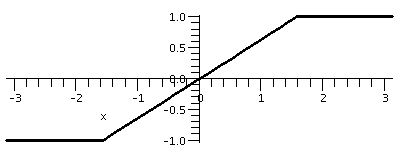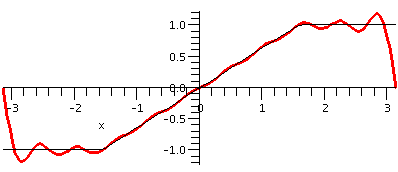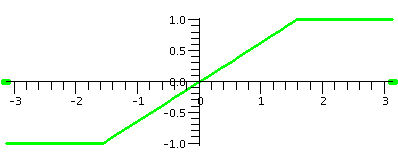The sum of the Fourier sine series of F (that is, the Fourier series of the odd extension of F) is equal to the original function except at the ends Pi and -Pi, where it averages the left and right behavior.

HOMEWORK
I strongly suggest that some time be spent in the next few days reviewing for the exam. I suggest doing some problems in 12.1-12.3. Also, you should read the review material.

 Confession

Monday, November 7

 Fourier series formulas
Orthogonal
Suppose that functions f(x) and g(x) are defined on the interval [a,b]. Then f(x) and g(x) are (mean square) orthogonal on [a,b] if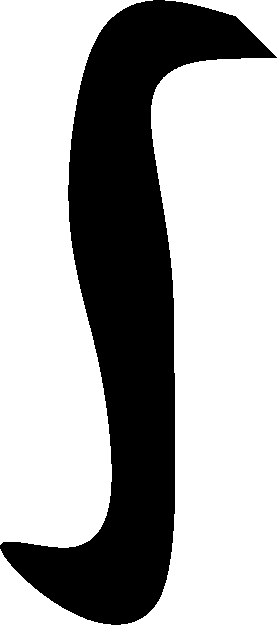abf(x)g(x) dx=0.
Comment I checked that this was the definition in the book, which it was. Sometimes people want to exclude the possibility that one of the functions is always 0. The "always 0" function is orthogonal to every function.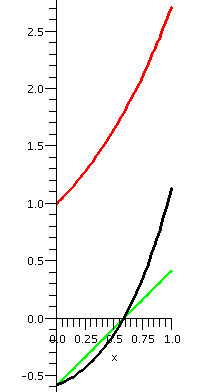Example
Here is an example suitable for a math course: suppose f(x)=ex and g(x)=x+C. Can you find C so that f(x) and g(x) are mean-square orthogonal on [0,1]?
Well, this means we want01ex(x+C) dx=0. Now ex(x+C)=xex+Cex has antiderivative xex-ex+Cex, so the definite integral (sigh, that stuff with ]x=0x=1) gives Ce-(-1+C). For this to be 0, we require C(e-1)+1=0 or C=-1/(e-1).

To the right are some pictures: the red curve is ex for x in the unit interval. The light green curve is x+C with C=-1/(e-1). Of course, the black curve is the product ex(x+C). It is supposed to be true that the area above the x-axis for the black curve is equal to the area below the x-axis for the black curve. That's what orthogonality means here.

Trig functions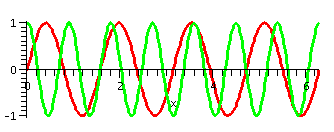The preceding example has little significance, as far as I know. But now we will verify something more interesting: the two functions sin(4x) and cos(7x) are orthogonal on [0,2Pi]. The first graph shown to the right has the graph of these two functions displayed on the interval mentioned. To me, the orthogonality of these two functions is not obvious.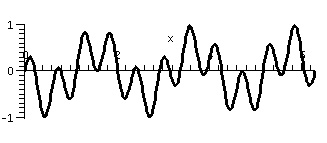The graph shows the prodcut, sin(4x)cos(7x), on the interval [0,2Pi]. Is it now obvious that the integral is equal to 0? Well, maybe ... but I think I would have to concentrate a bit. Instead, we'll verify the orthogonality algebraically. Recall from the first lecture:

Change t to -t in the formula, add, and divide by 2. The result is
cos(t)=(1/2)(ei(t)+e-i(t)).
If we substract them and divide by 2i the result is
sin(t)=(1/[2i])(ei(t)-e-i(t)).

How to antidifferentiate sin(4x)cos(7x)
Change t to 4x and write sin(4x)=(1/[2i])(ei(4x)-e-i(4x)). Change t to 7x and write cos(7x)=(1/2)(ei(7x)+e-i(7x)).
Then sin(4x)cos(7x)=
(1/2)(1/[2i])(ei(4x)-e-i(4x))(ei(7x)+e-i(7x))=
(1/2)(1/[2i])(ei(11x)-ei(3x)+ei(-3x)-ei(-11x)).
Now suppose that (INTEGER) is a non-zero integer, positive or negative. Then02Piei(INTEGER)x dx=1/(i(INTEGER))ei(INTEGER)x]02Pi. But when x=0 this is 1. And when x=2Pi(INTEGER)i, it is also 1 since sin((INTEGER))=0 and cos((INTEGER))=1.
Wow! So each of the four pieces of the sin(4x)cos(7x) integral evaluates to 0.

Something similar could be done for sin(4x) and sin(7x), and for cos(4x) and cos(7x). Many pairs of these functions are orthogonal.

Family of orthogonal functions
In fact, we have a family of orthogonal functions: {sin(nx),cos(mx)} where n is a positive integer and m is a non-negative integer. Every distinct pair of these functions is orthogonal. By :distinct" I mean that a function won't be orthogonal to itself. For example, sin(3x) multiplied by sin(3x) on [0,2Pi] doesn't have its integral equal to 0!
Note that we don't need negative n's and m's since sin(-3x)=-sin(3x), so02Pisin(-3x)sin(3x) dx isn't 0. Similarly for cos(-3x)=cos(3x).
In the family {sin(nx),cos(mx)} we don't really need the function sin(0x) since that is 0. We do, however, need the function cos(0x): that's 1.

Minimize the mean square energy
I remarked that the mean square norm, the integral of a function squared on an interval, was frequently identified as energy. So here is my task: given some function f(x) on [0,2Pi], consider the following function of, say, 7 variables:
E(a0,a1,a2,a3,b1,b2,b3)=02Pi(f(x)-(a0+a1cos(x)+a2cos(2x)+a3cos(3x)+b1sin(x)+b2sin(2x)+b3sin(3x)))2dx
This is a complicated function of seven (!) variables. Notice, please, that "x" is not a variable in E: it is internal to the integral. How can we minimize this function? This amounts to getting the best mean square approximation to f(x) by a trigonometric polynomial of the type given.

One reason to use squares is that they are differentiable. And the function E is certainly differentiable, although maybe it looks very complicated. Why should we expect or even hope that E has a minimum value? Well, since it is the integral of a square, E's value is at least 0. So certainly E is bounded below. It therefore can't run off to -infinity as the values of the parameters change.

The function E must have its minimum at a critical point, where all of its first derivatives are 0. Let's look at the first derivative of E with respect to b2:
dE/db2= d/db202Pi(STUFF)2dx=02Pi2STUFF(dSTUFF/db2) dx.
Since STUFF is f(x)-(a0+a1cos(x)+a2cos(2x)+a3cos(3x)+b1sin(x)+b2sin(2x)+b3sin(3x)) I know that d/db2 of it is just sin(2x). So we need to compute
202Pi(f(x)-(a0+a1cos(x)+a2cos(2x)+a3cos(3x)+b1sin(x)+b2sin(2x)+b3sin(3x)))sin(2x) dx. But we have shown that sin(2x) is orthogonal to 1 and cos(x) and cos(2x) and cos(3x) and sin(x) and sin(3x). So a bunch of terms (six of them!) "drop out" of this integral (they are all 0!) and we are left with
202Pi(f(x)-(b2sin(2x)))sin(2x) dx. This is 202Pi f(x)sin(2x)-b2sin(2x)sin(2x) dx.
When is this equal to 0? The 2 drops out, and this occurs when b2=02Pif(x)sin(2x) dx/02Pi(sin(2x))2dx.

The formulas
The best mean square approximation to f(x) on [0,2Pi] by a sum of trig polynomials occurs when
am=02Pif(x)cos(mx) dx/02Pi(cos(mx))2dx for m>=0
bn=02Pif(x)sin(nx) dx/02Pi(sin(nx))2dx for n>0.

The constants
We actually saw formulas like this before when we discussed orthonormal bases, and how to compute the coefficients for a linear combination in such a basis. The numbers02Pi(cos(mx))2dx and02Pi(sin(nx))2dx are normalization constants, and make the lengths of the appropriate vectors equal to 1 (they "normalize" the lengths).
Here I know that the normalization constants must be Pi. There is one exception. That is when m=0, and we have cos(0x)=1 for all x. Then the constant is 12=1 integrated over [2,2Pi], so it must be 2Pi.
Explanation Since sin2+cos2=1 and [0,2Pi] is a multiple of several full periods of sin(nx) and cos(mx), then, since integral of 1 over [0,2Pi] is 2Pi, and the shapes of sin2 and cos2 are the same, they each have integral equal to Pi.

The Fourier series of a function
If F(x) is a function defined on the interval [0,2Pi], define
an=(1/Pi)02PiF(x)cos(nx)dx
bn=(1/Pi)02PiF(x)sin(nx)dx
The Fourier series of F(x) is the infinite series of functions
a0/2+SUMN=1infinity(ancos(nx)+bnsin(nx))
where
an=(1/Pi)02PiF(x)cos(nx) dx for n integer, n>=0
bn=(1/Pi)02PiF(x)sin(nx) dx for n integer, n>0

Weird things to note
Well, these are weird but they are what's usual in the subject. Notice that the a0 term is divided by 2. That's because the normality constant for cos(0x) is 2Pi, not Pi. And also notice that the rest of the normalizing constants come off the formulas for the coefficients. In many standard linear algebra contexts, the darn formulas have the normalizations (those silly square roots) somehow in both the vectors and the coefficients of the vectors. Maybe what is done with Fourier series is more sensible.

You tell me how the Fourier series of a function relates to the function

I gave the class a handout. I wanted, in observation and discussion with students, to discover relationships (some subtle) between a function and its Fourier series (or, rather, since one can't add up all of any real infinite sum, the partial sums of the Fourier series): more heuristic stuff.
I would also like to have the Maple commands shown there available for you to copy, if you have the time and desire to experiment with them. Here they are:

• g:=n->(1/Pi)*int(F(x)*sin(n*x),x=0..2*Pi); Creates the sine coefficients.
• h:=n->(1/Pi)*int(F(x)*cos(n*x),x=0..2*Pi); Creates the cosine coefficients.
• Q:=N->h(0)/2+sum(h(n)*cos(n*x)+g(n)*sin(n*x),n=1..N); Adds things up and provides a linear combination of trig functions which is the Nth partial sum of the Fourier series of F.
• plot({F(x),Q(3)},x=0..2*Pi,thickness=3,scaling=constrained); Plots the function F(x) together with the third partial sum of its Fourier series.
Before any of these instructions are executed, a definition of F(x) is useful. The first function I investigated is (1/10)x2, which is defined by the command:
F:=x->(1/10)*x^2;
After this definition I checked to see if things were "working" by requesting Q(3):
```>Q(3);
2
2 Pi
----- + 2/5 cos(x) - 2/5 Pi sin(x) + 1/10 cos(2 x)
15

- 1/5 Pi sin(2 x) + 2/45 cos(3 x) - 2/15 Pi sin(3 x)```
Each coefficient is gotten by integrating by parts twice.

This F(x) and the 3rd partial
sum of its Fourier series
This F(x) and the 10th partial
sum of its Fourier series
This F(x) and the 20th partial
sum of its Fourier series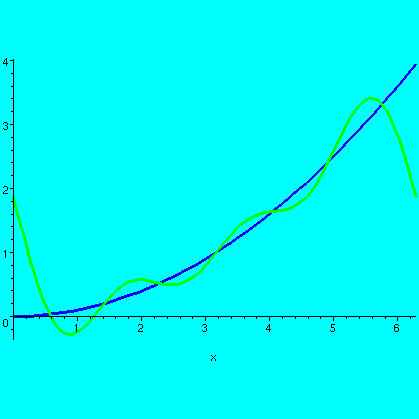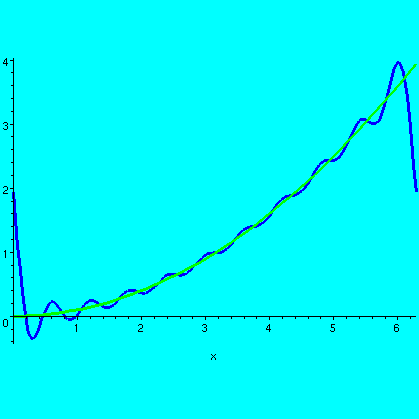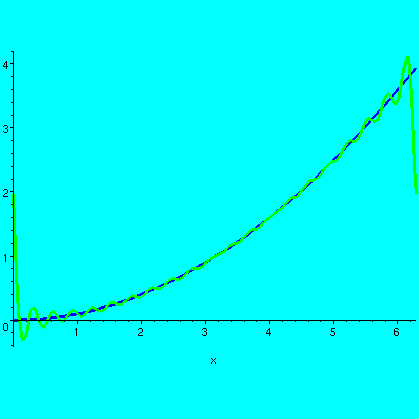The graphs of the Q(n)'s (the partial sums) get closer to the graph of F(x) as n increases.

What does closer mean? This turns out to be a rather difficult question, both theoretically and in practice.The pictures should show some of the difficulty. For example, you may want a function to be small on [a.b]. A very strict interpretation might be to have the values, f(x), very close to 0 for all x. But suppose you were really modelling some process which you expected to sample, somehow "randomly", on the interval, a few times (10 or 100 or ...). Maybe you would be happy enough controlling the average distance to 0. So things are complicated.

In the pictures of our function F(x) and various partial sums, inside the interval the partial sums are getting close to the values of the function. At the end points (0 and 2Pi) they aren't getting close ... what the heck. Also, if you look really closely at the graphs, you can see tiny bumps near the "ends" which represent some complicated phenomena. Well, one thing at a time.

What the Fourier series sees...
We get the Fourier coefficients by integrating the product of a sine or cosine on [0,2Pi] (the solid green curve) by our function F(x) (the solid magenta [?]) curve). One point of view is that everything goes on inside the shaded box. But the trig function goes on forever, and it is periodic with period 2Pi. To the trig function, our F(x) might as well be "extended" with period 2Pi to the left and to the right forever. Notice that the trig function will try at, say, 0, to approximate the values from both the left and right of the extended F(x). This extended F(x) has a jump discontinuity at 0, and the trig function, in trying its approximation, settles on being halfway between the ends of the jump. This is the collection of black dots in the picture at half the height of F(x) at x=2Pi.The partial sums of the Fourier series try very hard to get close to F(x). If F is continuous at x, then they will converge to F(x). If F has a jump discontinuity at x, then they will converge to the average (really!) of the left and right hand limits of F at x (the middle of the jump).

Gibbs: the overshoot
J. Willard Gibbs received the first U.S. doctorate in engineering in 1863. He saw that at a jump discontinuity, there is always an overshoot of about 9% in the Fourier series. On the top side, the overshoot is above, and on the bottom side, below. These bumps get narrower and closer to the jump, but they never disappear!

A Heaviside example
The next example on the handout was U(x-{Pi/2}), the Heaviside step or jump at Pi/2. This function is 0 to the left of Pi/2 and is 1 to the right of Pi/2. In Maple, the following formula describes the function: F:=x->piecewise(x<Pi/2,0,1);
Here are the pictures for this function.

This F(x) and the 3rd partial
sum of its Fourier series
This F(x) and the 10th partial
sum of its Fourier series
This F(x) and the 20th partial
sum of its Fourier series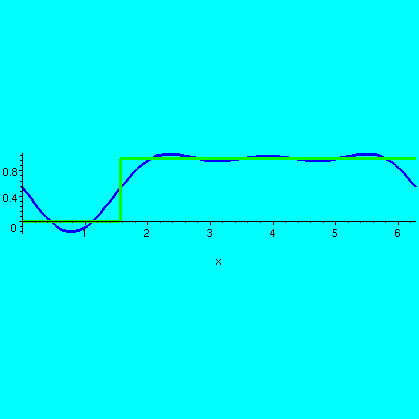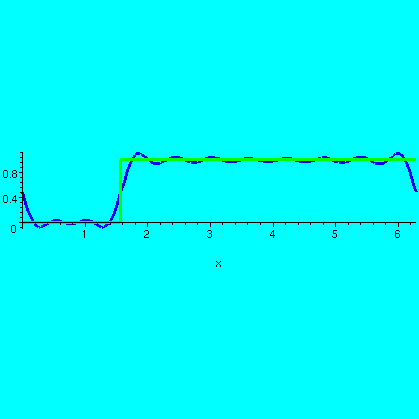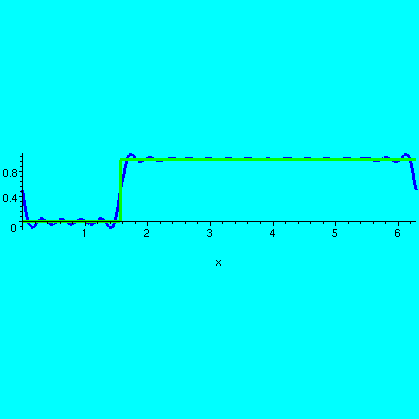I hope you see that the partial sums detect two jump discontinuities, one at Pi/2, certainly, but another one at 0=2Pi (well, they are the same numbers to sine and cosine) as well!

Taylor and Fourier compared
I tried to take a fairly random function defined by a fairly simple formula: F(x)=sqrt(16+x2+x3). Then I had Maple create the degree 12 Taylor polynomial for F(x) at x=0. I also had Maple create the Fourier series summed up to the n=6 terms in both sine and cosine: this is what is defined as Q(6) above. I admit that the computation of Q(6) took more time than the computation of the Taylor polynomial, but not a great deal more time. Here are three pictures.
 This is the Taylor polynomial compared with the function in the interval [0,3]. For much of the left-hand portion of this interval, the Taylor polynomial and the function graph overlay one another.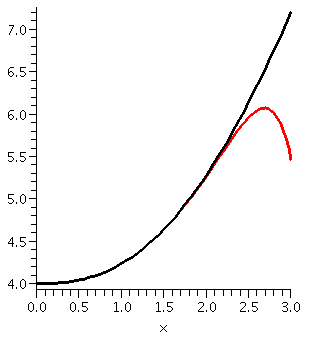Now the Taylor polynomial and the function are shown on all of [0,2Pi]. Please note the scale on the vertical axis. There is enormous discrepancy between the function and the polynomial for much of the domain.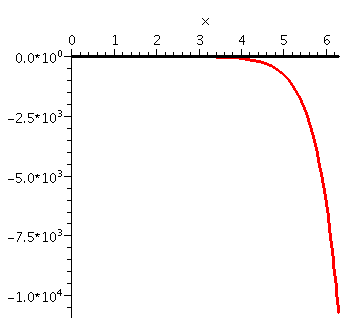Here is the Fourier approximation together with the function on all of [0,2Pi]. There is certainly some deviation, but the deviation is controlled and only affects a small part of the domain. On average these functions are rather close.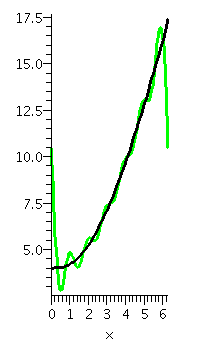The exam
The exam will cover our work on linear algebra and sections 12.1-12.3 of the textbook, which we will discuss this week.

Textbook problems
We won't have time to give and get back graded homework. But the fine students listed below associated with the textbook homework problems indicated (from the syllabus) have agreed to try to write solutions which I will scan and put on the web.

12.1: 3, Mr. Sequeira
12.1: 7, Ms. Launay
12.1: 17, Mr. Weinstein
12.2: 1, Ms. Tagle
12.2: 5, Ms. Rose
12.2: 9, Mr. Clark
12.2: 15, Mr. Boege
12.2: 17, Mr. Mostiero

Earn 5 points
You can earn 5 points towards your score on the next exam by answering some questions. The rules are on that page.

HOMEWORK
You should read sections 12.1-12.3 of the text. Today we covered much of 12.1 and 12.2, and I hope to discuss 12.3 on Thursday. I would suggest that you try several of the homework problems assigned in 12.1 and 12.2, and even look at the other textbook problems and consider if you can do them.